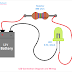Hey, in this article we are going to see the LED Connection Diagram and Wiring Procedure in both Series and Parallel combination. LED means Light Emitting Diode is a semiconductor device that emits light energy from its junction when a forward voltage is applied across it. Nowadays, LED light is the most usable light in our homes, offices. LED is a very low power-consuming device. The main advantages of LED are low power loss, high efficiency, more reliability low operating voltage, and current, etc. Also, LEDs cause very low energy pollution. LED lights are comes in the form of bulbs, LED Strips, Projection Lights, Holders, etc.

## A Simple LED Connection

Here, you can see a simple connection of LED with a battery.

Here, the LED has a rating of 3.5V, 10mA. So, to operate the LED properly, we have to apply a 3.5V DC supply across the LED. And the full load current of the LED is 10mA means it can consume a maximum of 10mA current. Here, we have taken a 12V battery. So we need to connect a resistor in series with the LED and Battery, otherwise, the LED will be burned. Now, the question is how to select the value of the resistor for the LED. It is very simple. The formula for finding the value of the resistor is Resistor value = (Battery Voltage - LED Voltage)/LED Current.

So, here the battery voltage is 12V, LED Voltage is 3.5V, and the LED Current is 10mA or 0.001A. Now the resistor value will be R = (12-3.5)/0.001 = 850ohm. It is the general method to find the value of the resistor. But we can use a resistor that has a rating of 750ohm to 850ohm. Remember that, if you use a resistor more than 850ohm, the brightness of the LED will be decreased and if you use a resistor less than 750ohm the LED may burn.

While connecting a LED with a power supply, first consider the voltage and current rating of the LED and voltage of the power supply. Then calculate the value of the resistor. Remember, that the LED will work only when it is connected in forward bias only, which means its positive terminal is to be connected to the positive terminal of the battery or power source and the negative terminal of the LED is to be connected to the negative terminal of the battery or power source. Also, remember that LED will work properly with a DC power supply only, if you connect a LED with an AC power supply, it will be flickering according to the frequency of the AC power supply.

## Multiple LED Wiring in Series Connection

Here, you can see the diagram to understand how to connect LED in series.

The battery voltage is 12V. As the LEDs are connected in series, so the total voltage of the circuit will be (2.75 + 2.75 + 2.75 + 2.75) = 11V. So the circuit can be connected to the battery without connecting any resistor. If you connect two or three LEDs in series then you must connect a resistor, otherwise, LEDs will be burned. To find out the resistor value, make the sum voltage of each LEDs and take the maximum current rating of the any one LED.

## Multiple LED Wiring in Parallel Connection

Here, you can see the diagram to understand how to connect LED in parallel.

In a parallel connection, the voltage across each LED will be the same. In the above diagram, all the LEDs have the same voltage and current rating. That is why resistors connected to all the LEDs are have the same value. If you connect LEDs having different voltage and current ratings, calculate the resistor value individually and connect to the circuit.

LED Connection Diagram and Wiring Procedure - Series, ParallelReviewed by Author on November 03, 2021 Rating: 5